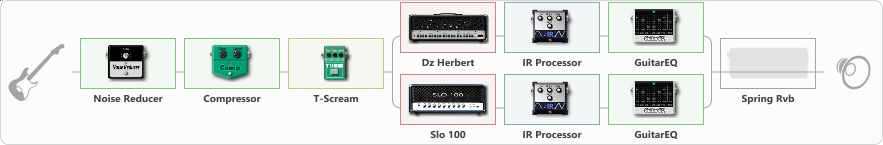# METAL FOR YOU

Discussion in 'ToneLib-GFX presets' started by Itadachi, Dec 27, 2022.

Tags:
1. METAL FOR YOU
Preset name: METAL FOR YOU

Effects chain:Effect: "Noise Reducer" (Dynamics / Filter), active - "yes"
{

"Sens" = 63
"Mode" = Hard
}

Effect: "Compressor" (Dynamics / Filter), active - "yes"
{

"Sense" = 24
"Level" = 66
}

Effect: "T-Scream" (Overdrive / Distortion), active - "yes"
{

"Drive" = 38
"Tone" = 80
"Level" = 79
}

Effect: "Splitter" (Dynamics / Filter)
{

"A-Bypass" = Off
"A-Pan" = -22
"A-Level" = 55
"B-Bypass" = Off
"B-Pan" = 100
"B-Level" = 55
"Width" = 0
'A' branch:
{

Effect: "Dz Herbert" (Amp simulators), active - "yes"
{

"Gain" = 51
"Bass" = 52
"Middle" = 57
"Treble" = 44
"Presence" = 50
"Master" = 81
"Level (dB)" = 8
}
Effect: "IR Processor" (Cabinets), active - "yes"
{

"IR" = Cab IR Sample2
"Low Cut (Hz)" = 0
"Hi Cut (kHz)" = 17.5
"Mix" = 97
"Level (dB)" = 0
}
Effect: "GuitarEQ" (Dynamics / Filter), active - "yes"
{

"160 Hz" = 0
"400 Hz" = 1
"800 Hz" = 0
"1.6 kHz" = 3
"3.2 kHz" = 3
"6.4 kHz" = 0
"12 kHz" = 0
"Level (dB)" = 0
}
}
'B' branch:
{

Effect: "Slo 100" (Amp simulators), active - "yes"
{

"Gain" = 49
"Bass" = 39
"Middle" = 58
"Treble" = 63
"Presence" = 50
"Master" = 56
"Level (dB)" = 6
}
Effect: "IR Processor" (Cabinets), active - "yes"
{

"IR" = Cab IR Sample2
"Low Cut (Hz)" = 24
"Hi Cut (kHz)" = 20.0
"Mix" = 98
"Level (dB)" = 0
}
Effect: "GuitarEQ" (Dynamics / Filter), active - "yes"
{

"160 Hz" = -5
"400 Hz" = 1
"800 Hz" = -3
"1.6 kHz" = 0
"3.2 kHz" = 0
"6.4 kHz" = 0
"12 kHz" = 0
"Level (dB)" = -1
}
}
}

Effect: "Spring Rvb" (Reverberation), active - "no"
{

"Time" = 5.1
"PreDelay" = 8
"LoDamp" = 16
"HiDamp" = 20
"Mix" = 25
}

Note: You will need to download and install the ToneLib-GFX software to use the preset.

#### Attached Files:

• ###### METAL_FOR_TOU.tlgfx
File size:
6 KB
Views:
4,536
Last edited: Feb 26, 2023